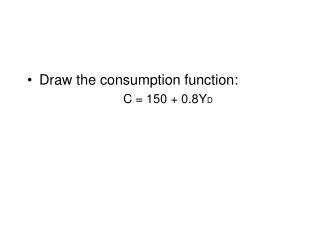DownloadDownload PresentationDraw the consumption function: C = 150 + 0.8Y D

# Draw the consumption function: C = 150 + 0.8Y D

Download Presentation## Draw the consumption function: C = 150 + 0.8Y D

- - - - - - - - - - - - - - - - - - - - - - - - - - - E N D - - - - - - - - - - - - - - - - - - - - - - - - - - -
##### Presentation Transcript

1. Draw the consumption function: C = 150 + 0.8YD

2. Draw the consumption function: C = 150 + 0.8YD At what level of income do households begin to save? By how much does consumption increase when income rises 200? 750 By 160 to 910

3. If the consumption function is: C = 200 + 0.9YD What does the saving function look like? What is the rate of desired saving when disposable income equals 500 1000 2000 S = -200 + 0.1YD -150 -100 0

4. Compute 4 rounds of multiplier effects when autonomous investment increases by 100 million in a closed, private economy (no foreign trade nor government) and MPC = 90%.

5. 1,000 million or 1 billion What will be the final cumulative impact on spending?

6. Suppose the consumption function is: C = 400 + 0.8YD By how much will aggregate demand at current prices shift initially with -A 50 billion increase in government purchases? -A 50 billion tax cut -A 50 billion increase in income transfers 50 billion 40 billion 40 billion Multiplier = 1 _ 1-MPC

7. Suppose the consumption function is: C = 400 + 0.8YD • What will be the cumulative AD shift for • A 50 billion increase in government purchases? • A 50 billion tax cut • A 50 billion increase in income transfers 250 billion 200 billion 200 billion Multiplier = 1 _ 1-MPC